Question

Please do exercise 129: Exercise 128: Define r:N + N by r(n) = next(next(n)). Let f:N...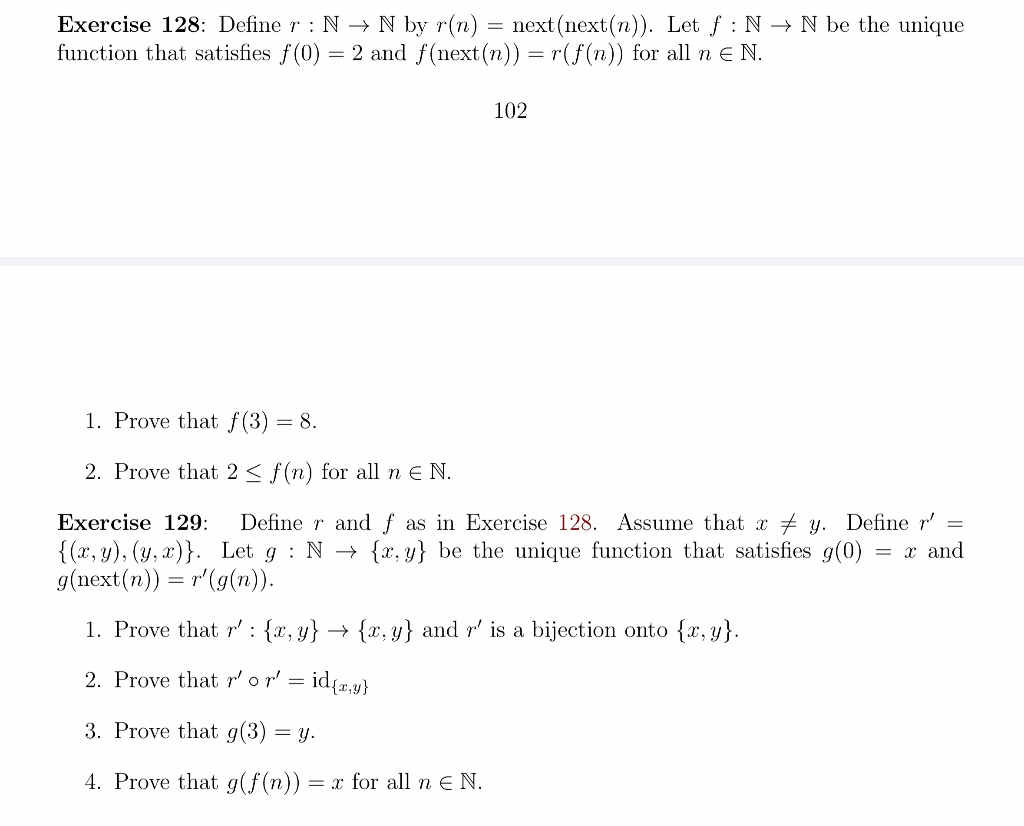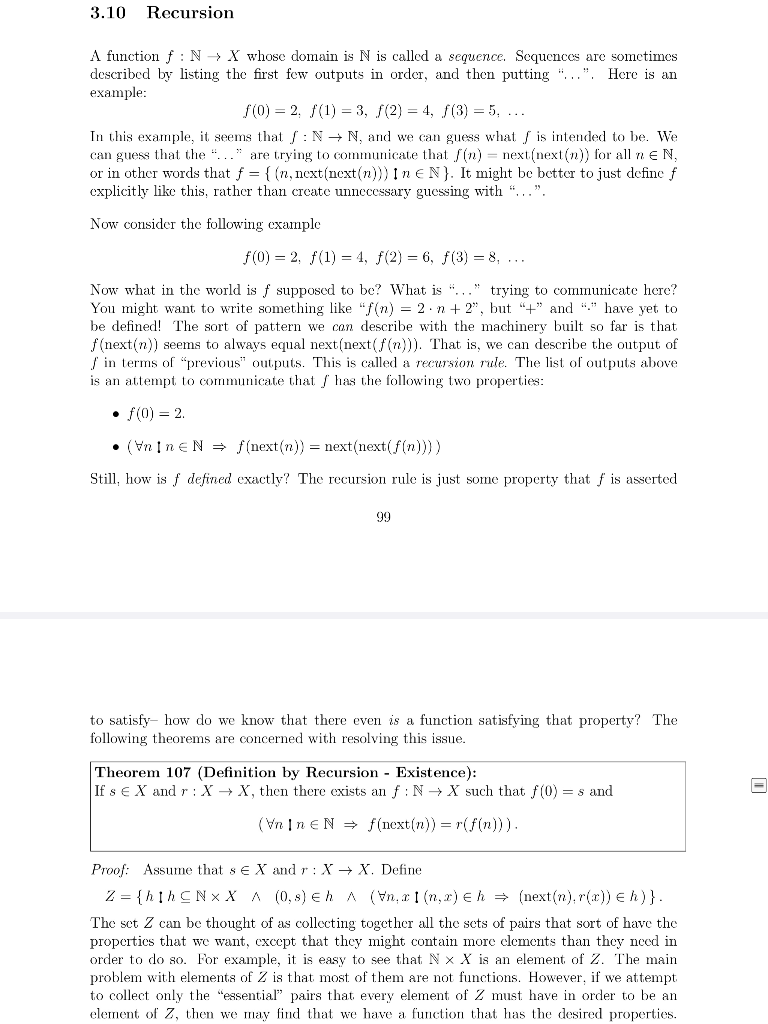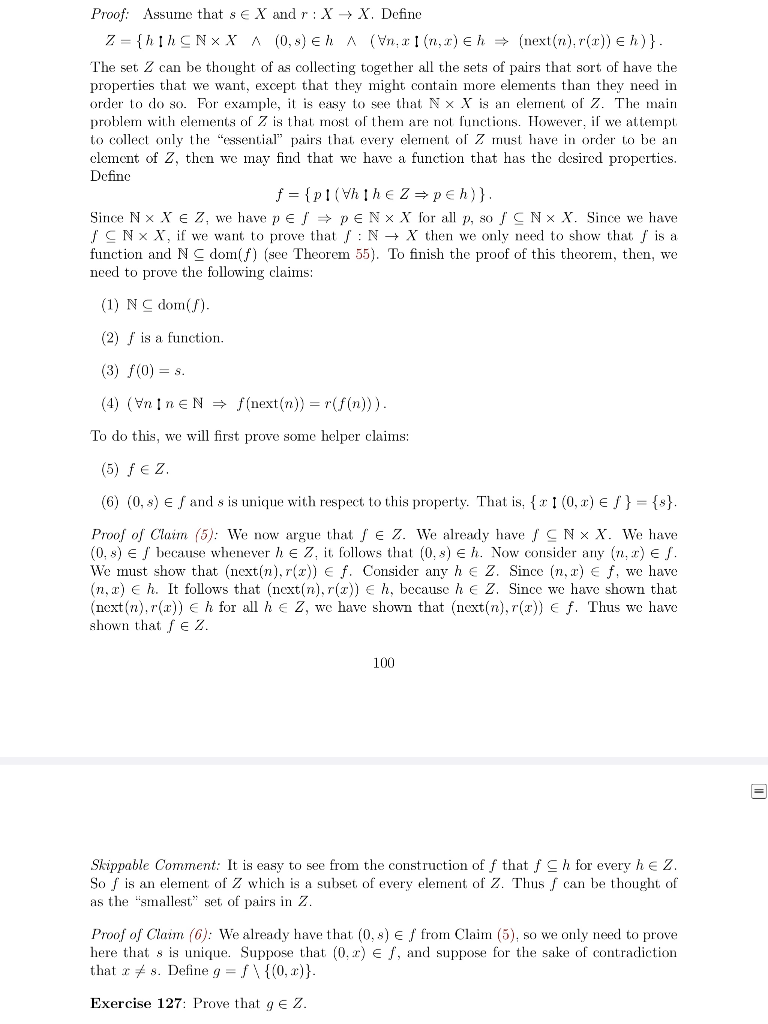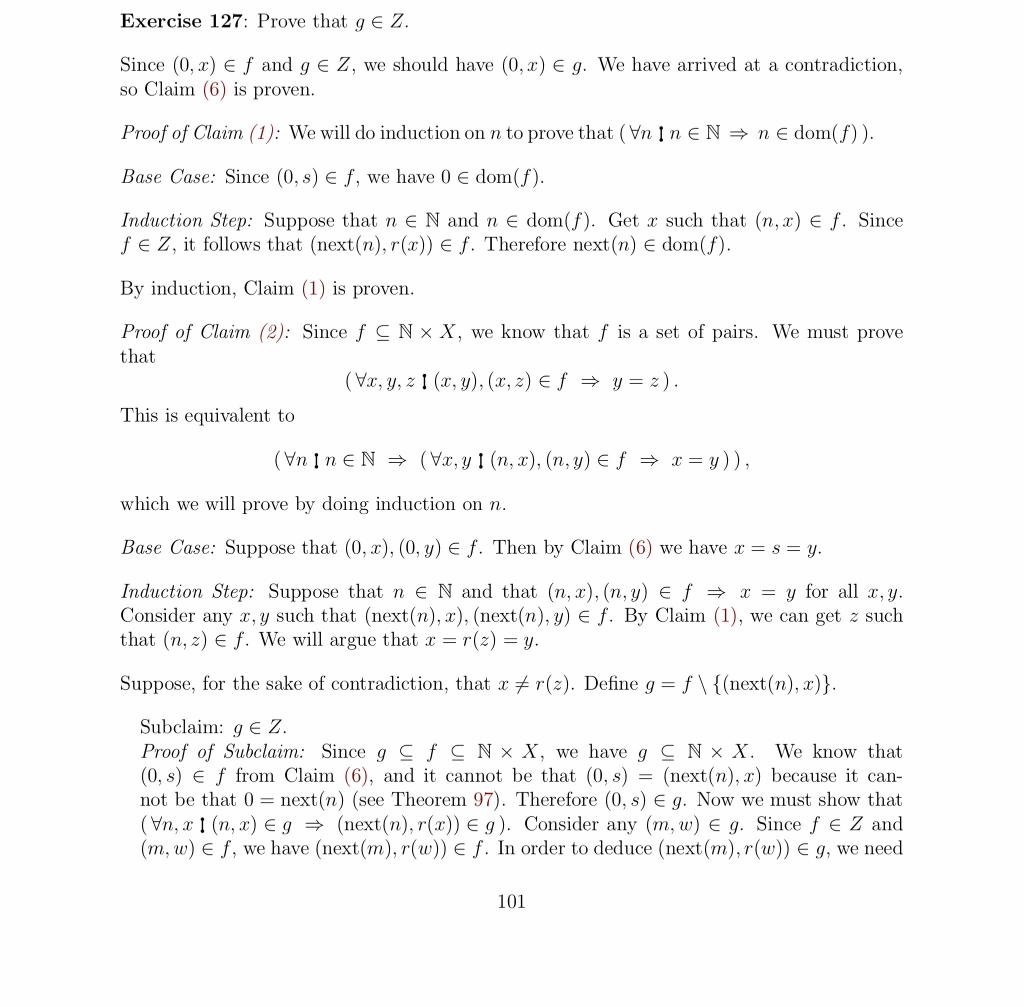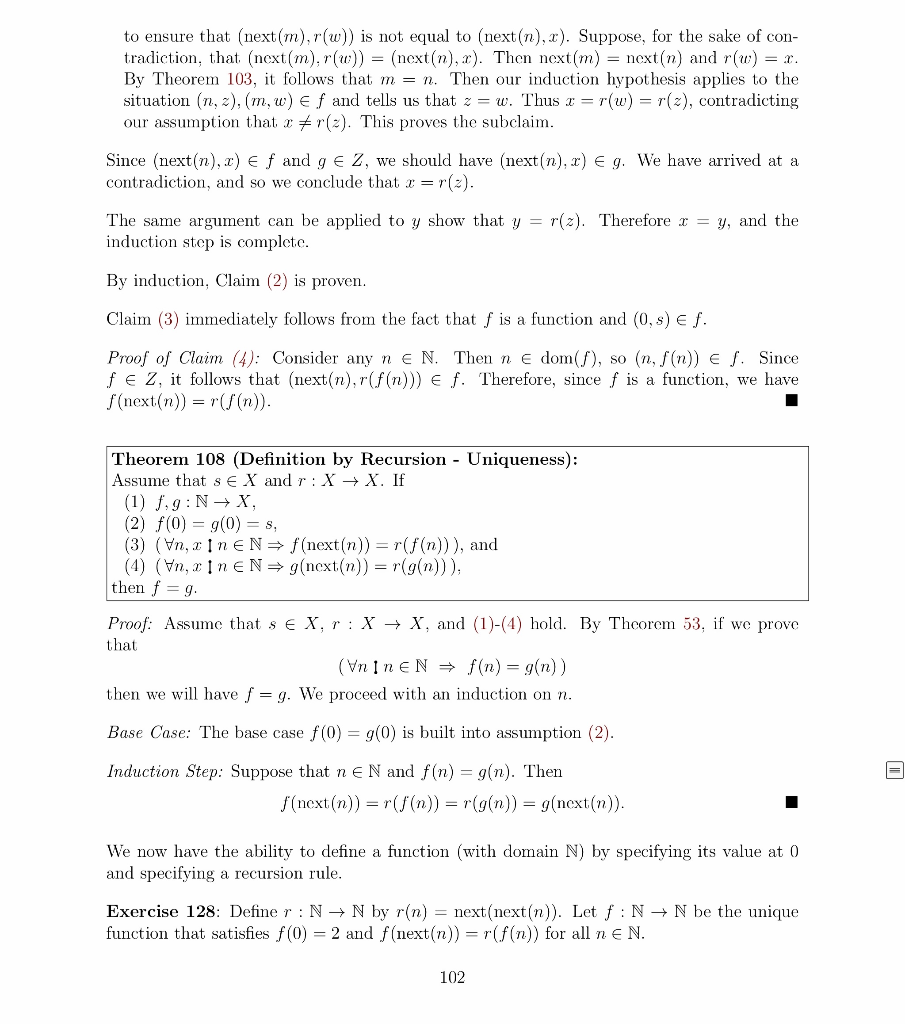Exercise 128: Define r:N + N by r(n) = next(next(n)). Let f:N → N be the unique function that satisfies f(0) = 2 and f(next(n)) =r(f(n)) for all n E N. 102 1. Prove that f(3) = 8. 2. Prove that 2 <f(n) for all n E N. Exercise 129: Define r and f as in Exercise 128. Assume that x + y. Define r' = {(x,y),(y,x)}. Let g:N + {x,y} be the unique function that satisfies g(0) = x and g(next(n)) = r' g(n)). 1. Prove that r': {x, y} + {x,y} and r' is a bijection onto {x, y}. 2. Prove that r'on' = idęz,y} 3. Prove that g(3) = y. 4. Prove that gif(n)) = x for all n E N.
3.10 Recursion A function f:N X whose domain is N is called a sequence. Sequences are sometimes described by listing the first few outputs in order, and then putting "...". Here is an example: f(0) = 2. f(1) = 3, (2) = 4, f(3) = 5, ... In this example, it seems that /:N + N, and we can guess what is intended to be. We can guess that the "..." are trying to communicate that lin) = next next(n)) for all n EN, or in other words that f = { (n.next(next(n)))INEN}. It might be better to just define f cxplicitly like this, rather than create unnecessary guessing with "...". Now consider the following cxample f(0) = 2. f(1) = 4, F(2) = 6, f(3) = 8, ... Now what in the world is f supposed to be? What is "..." trying to communicate here? You might want to write something like "f(n) = 2.n + 2", but "+" and "." have yet to be defined! The sort of pattern we can describe with the machinery built so far is that f(next(n)) seems to always equal next(next(f(n))). That is, we can describe the output of / in terms of "previous" outputs. This is called a recursion rule. The list of outputs above is an attempt to communicate that / has the following two properties: • f(0) = 2. . (Enine N = f(next(n)) = next(next( f(n)))) Still, how is / defined exactly? The recursion rule is just some property that is asserted 99 to satisfy- how do we know that there even is a function satisfying that property? The following theorems are concerned with resolving this issue. Theorem 107 (Definition by Recursion - Existence): If s E X and r : X X , then there exists an f:N → X such that f(0) = 8 and (Vn in EN → f(next(n)) = rfin))). Proof: Assume that s e X and r : X + X. Define Z = {hih CNXX A (0,8) eh A (Hn. 11 (n,r) eh → (next(n).r(:)) € h)}. The set Z can be thought of as collecting together all the sets of pairs that sort of have the properties that we want, except that they might contain more clements than they need in order to do so. For example, it is easy to see that N X X is an element of Z. The main problem with elements of Z is that most of them are not functions. However, if we attempt to collect only the "essential” pairs that every element of 2 must have in order to be an element of Z, then we may find that we have a function that has the desired properties.
Proof: Assume that s e X and r :X + X. Define 7 = {hih CNX X A (0,8) Eh A (Vn, (n,) eh → (next(n).rc)) eh)}. The set 2 can be thought of as collecting together all the sets of pairs that sort of have the properties that we want, except that they might contain more elements than they need in order to do so. For example, it is easy to see that N X X is an element of Z. The main problem with elements of Z is that most of them are not functions. However, if we attempt to collect only the essential" pairs that every element of z must have in order to be an clement of 2, then we may find that we have a function that has the desired properties. Define f = {pi (Whihez peh)}. Since NX XEZ, we have pe → PENxX for all p, so / CNX X. Since we have / CNX X, if we want to prove that /:N + X then we only need to show that is a function and NC domif) (see Theorem 55). To finish the proof of this theorem, then, we need to prove the following claims: (1) NC dom(). (2) / is a function. (3) f(0) = s. (4) (nin eN = f(next(n)) = r(f(n))). To do this, we will first prove some helper claims: (5) fez (6) (0,5) € / and s is unique with respect to this property. That is, 11 (0,) € / } = {s}. Proof of Claim (5): We now argue that / E Z. We already have / CNX X. We have (0.5) € / because whenever he Z, it follows that (0.) Eh. Now consider any (n.) € /. We must show that (next(n), r(2)) Ef. Consider any h e Z. Since (n,r) Ef. we have (n,r) eh. It follows that (nextín),r()) Eh, because he Z. Since we have shown that next(n).r(x)) h for all he Z, we have shown that next(n), 7()) Ef. Thus we have shown that fe 2. 100 Skippable Comment: It is easy to see from the construction of f that f Ch for every he Z. So f is an element of Z which is a subset of every element of Z. Thus can be thought of as the "smallest" set of pairs in Z. Proof of Claim (6): We already have that (0, ) ef from Claim (5), so we only need to prove here that s is unique. Suppose that (0.2) Eſ, and suppose for the sake of contradiction that s. Define g = /\ {(0, )}. Exercise 127: Prove that gez.
Exercise 127: Prove that ge Z. Since (0,x) ef and g € Z, we should have (0,2) Eg. We have arrived at a contradiction, so Claim (6) is proven. Proof of Claim (1): We will do induction on n to prove that (VnIn EN →n e dom(f)). Base Case: Since (0,) Ef, we have 0 € dom(f). Induction Step: Suppose that n e N and n e dom(\$). Get x such that (n,x) e f. Since fe Z, it follows that (next(n), r(c) Ef. Therefore next(n) e dom(f). By induction, Claim (1) is proven. Proof of Claim (2): Since f CNⓇ X, we know that f is a set of pairs. We must prove that (Vc, y, z (1, 2), (3, 4) + f = g = ). This is equivalent to (Vnin EN → (Vr, yi(n,x),(n,y) e f = x= y)), which we will prove by doing induction on n. Base Case: Suppose that (0,x),(0,y) e f. Then by Claim (6) we have x = s = y. Induction Step: Suppose that n e N and that (n,x),(n,y) e f = x = y for all x,y. Consider any x, y such that (next(n), x), (next(n), y) e f. By Claim (1), we can get z such that (n,r) Ef. We will argue that x = r() = y. Suppose, for the sake of contradiction, that x +r(). Define g=f\{(next(n), x)}. Subclaim: 9 € Z. Proof of Subclaim: Since g çf Ç N * X, we have g S N X. We know that (0,s) e f from Claim (6), and it cannot be that (0,5) = (next(n), x) because it can- not be that 0 = next(n) (see Theorem 97). Therefore (0, 3) eg. Now we must show that (Vn, x 1 (n,x) Eg → (next(n), r(2)) Eg). Consider any (m, w) E g. Since f e Z and (m, w) Eſ, we have (next(m), r(w)) Ef. In order to deduce (next(m), r(w)) Eg, we need 101
to ensure that (next(m), r(w)) is not equal to (next(n), x). Suppose, for the sake of con- tradiction, that (next(m),r()) = (next(n), r). Then next(m) = next(n) and r(w) = x. By Theorem 103, it follows that m = n. Then our induction hypothesis applies to the situation (1, 2). (m, w) ef and tells us that z = w. Thus c = r(w) = r(2), contradicting our assumption that I +r(:). This proves the subclaim. Since (next(n), c) ef and g € 2, we should have (next(n), x) Eg. We have arrived at a contradiction, and so we conclude that I=r(2). The same argument can be applied to y show that y = r(z). Therefore r = y, and the induction step is complete. By induction, Claim (2) is proven. Claim (3) immediately follows from the fact that f is a function and (0,) ef. Proof of Claim (4): Consider any n e N. Then n E dom(S), so (n, f(n)) € /. Since fe Z, it follows that (next(n),r(f(n))) Ef. Therefore, since f is a function, we have f(next(n)) = r(/(n)). Theorem 108 (Definition by Recursion - Uniqueness): Assume that se X and r:X + X. If (1) fig:N + X, (2) f(0) = g(0) = S, (3) (Vn, In EN= f(next(n)) = rf(n))), and (4) (n,xin EN= g(next.(n)) = r(g(n))), then f = g. Proof: Assume that sex, r :X + X, and (1)-(4) hold. By Theorem 53, if we prove that (VineN + f(n) = g(n)) then we will have f = g. We proceed with an induction on n. Base Case: The base case f(0) = g(0) is built into assumption (2). Induction Step: Suppose that ne N and f(n) = g(n). Then f(next(n)) = rf(n)) = r(g(n)) = g(next(n)). We now have the ability to define a function (with domain N) by specifying its value at 0 and specifying a recursion rule. Exercise 128: Define r:N + N by r(n) = next(next(n)). Let f:N + N be the unique function that satisfies f(0) = 2 and f(next(n)) = r f(n)) for all n E N. 102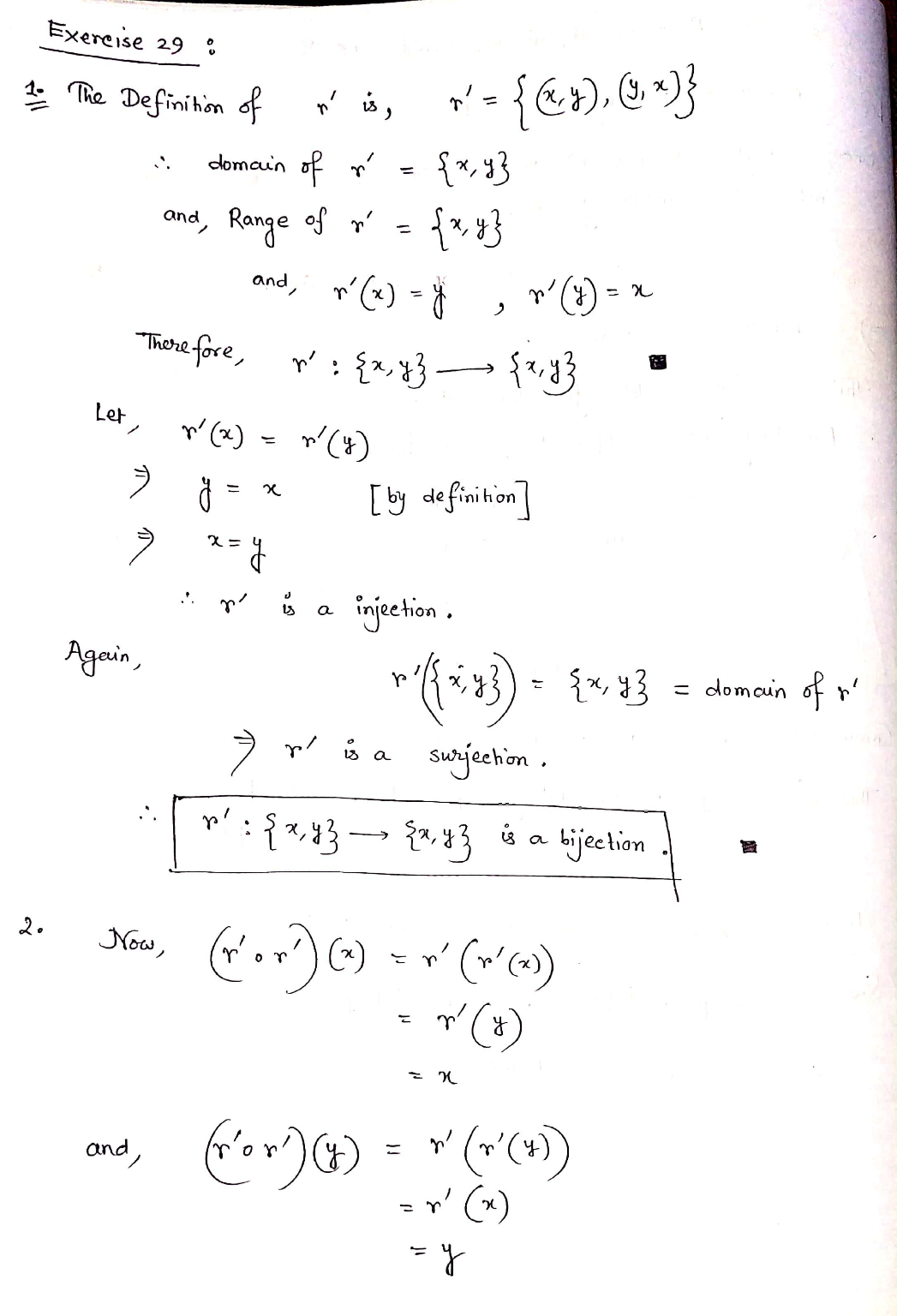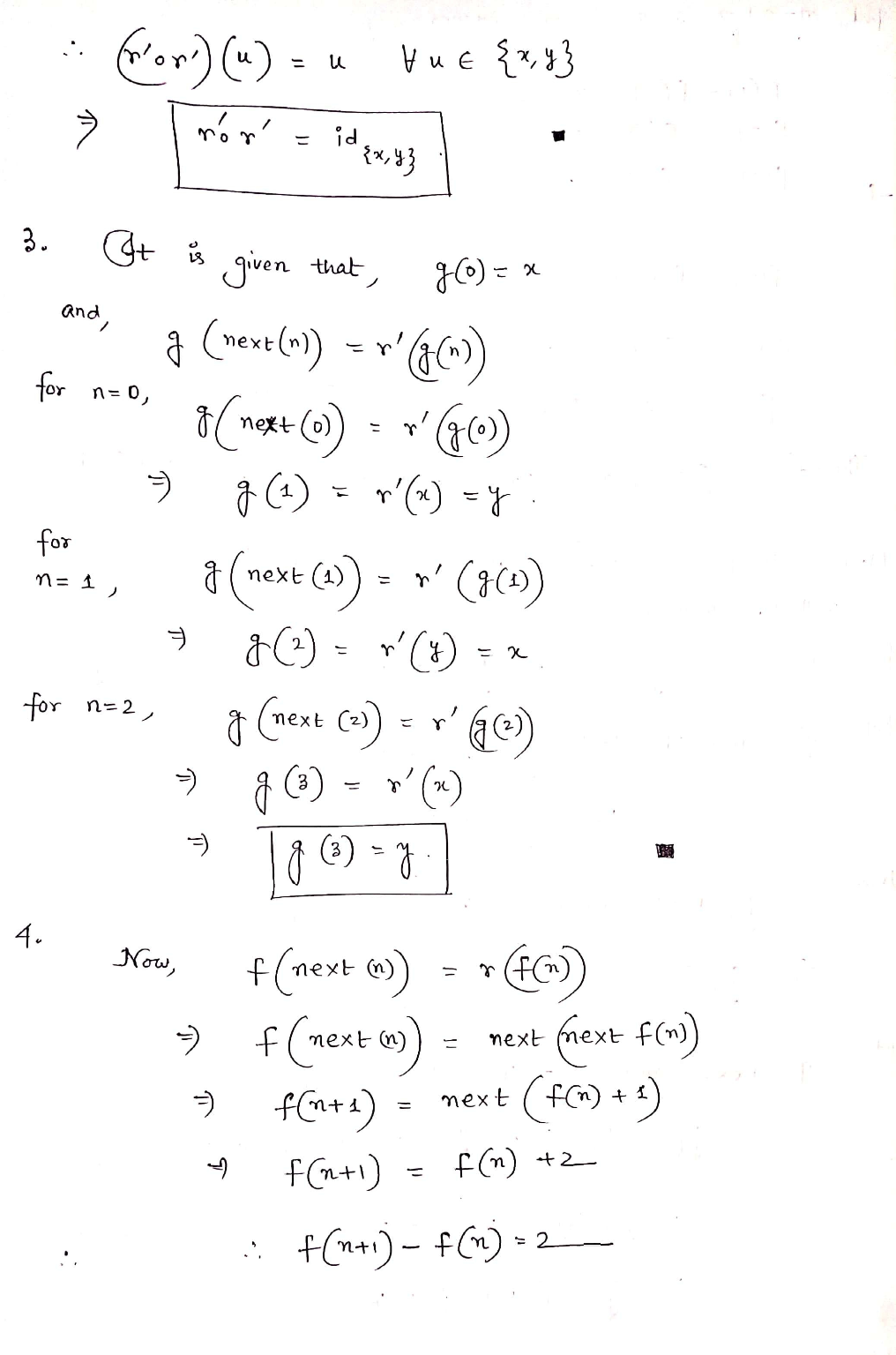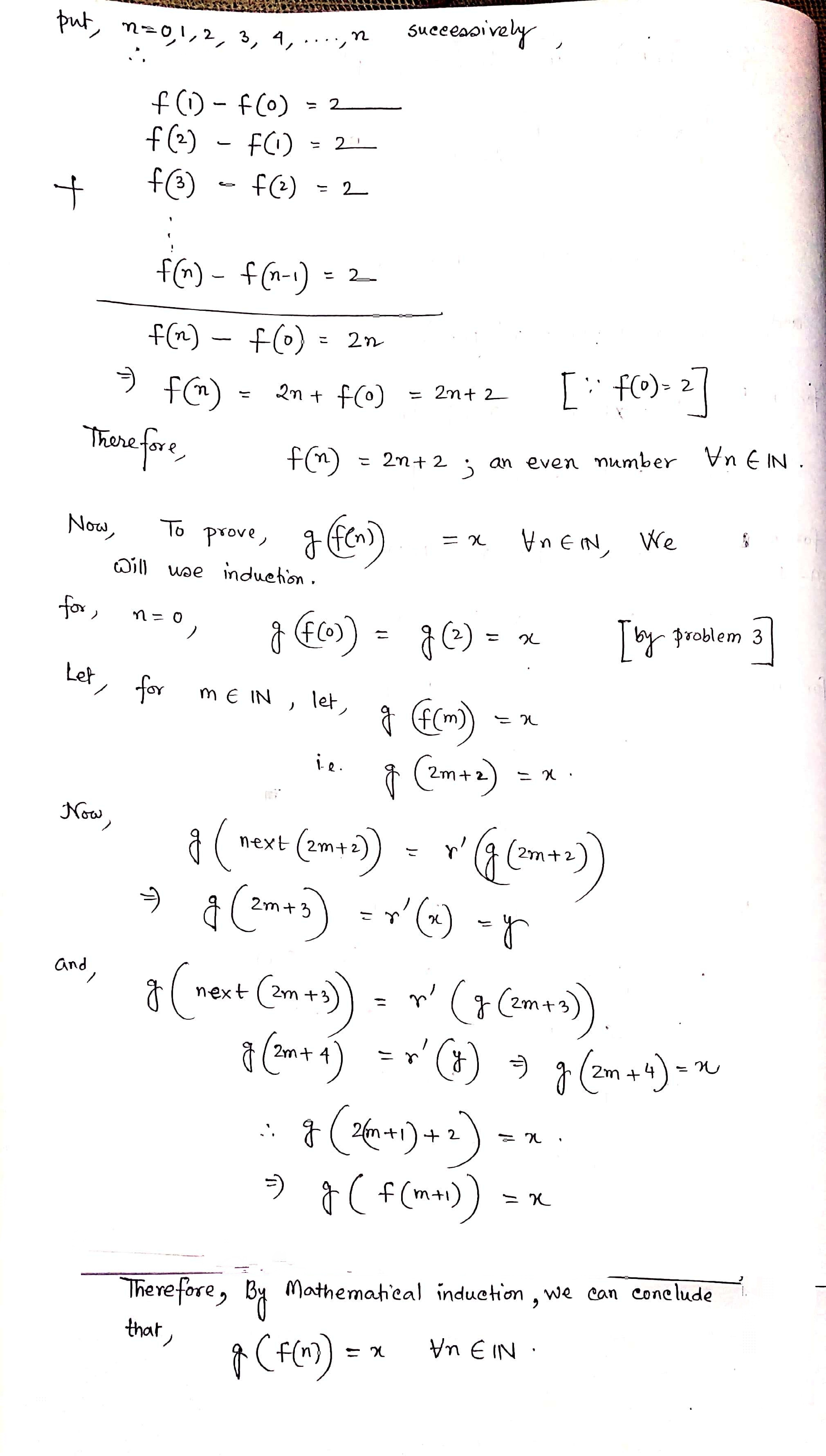Feel free to ask any doubts in comment section. Thank you sir/ma'am. ??

Earn Coins

Coins can be redeemed for fabulous gifts.

Similar Homework Help Questions
• 4. Define a function f:N → Z by tof n/2 if n is even 1-(n +...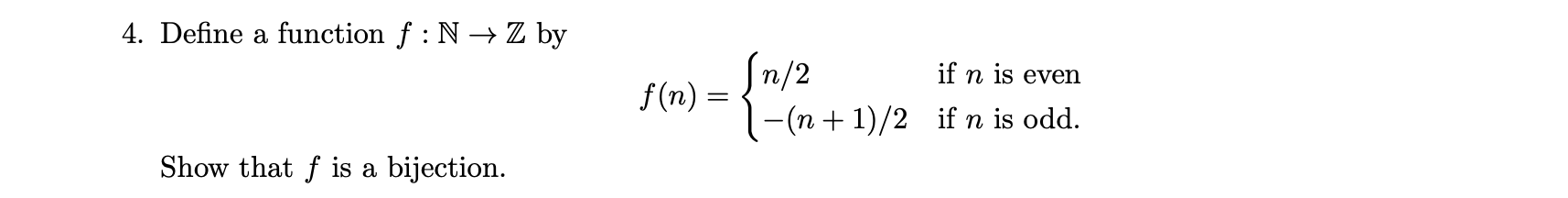4. Define a function f:N → Z by tof n/2 if n is even 1-(n + 1)/2 if n is odd. f(n) = Show that f is a bijection. 11 ] 7. Let X = R XR and let R be a relation on X defined as follows ((x,y),(w,z)) ER 4 IC ER\ {0} (w = cx and z = cy.) Is R reflexive? Symmetric? Transitive? An equivalence relation? Explain each of your answers. Describe the equivalence classes [(0,0)]R and...

• 5. Let A = P(R). Define f : R → A by the formula f(x) =...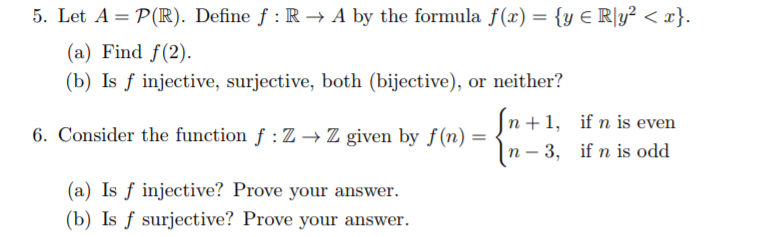5. Let A = P(R). Define f : R → A by the formula f(x) = {y E RIy2 < x). (a) Find f(2). (b) Is f injective, surjective, both (bijective), or neither? Z given by f(u)n+l, ifn is even n - 3, if n is odd 6. Consider the function f : Z → Z given by f(n) = (a) Is f injective? Prove your answer. (b) Is f surjective? Prove your answer

• Suppose f:N → N satisfies the recurrence f(n+1) = f(n) 7. Note that this is not...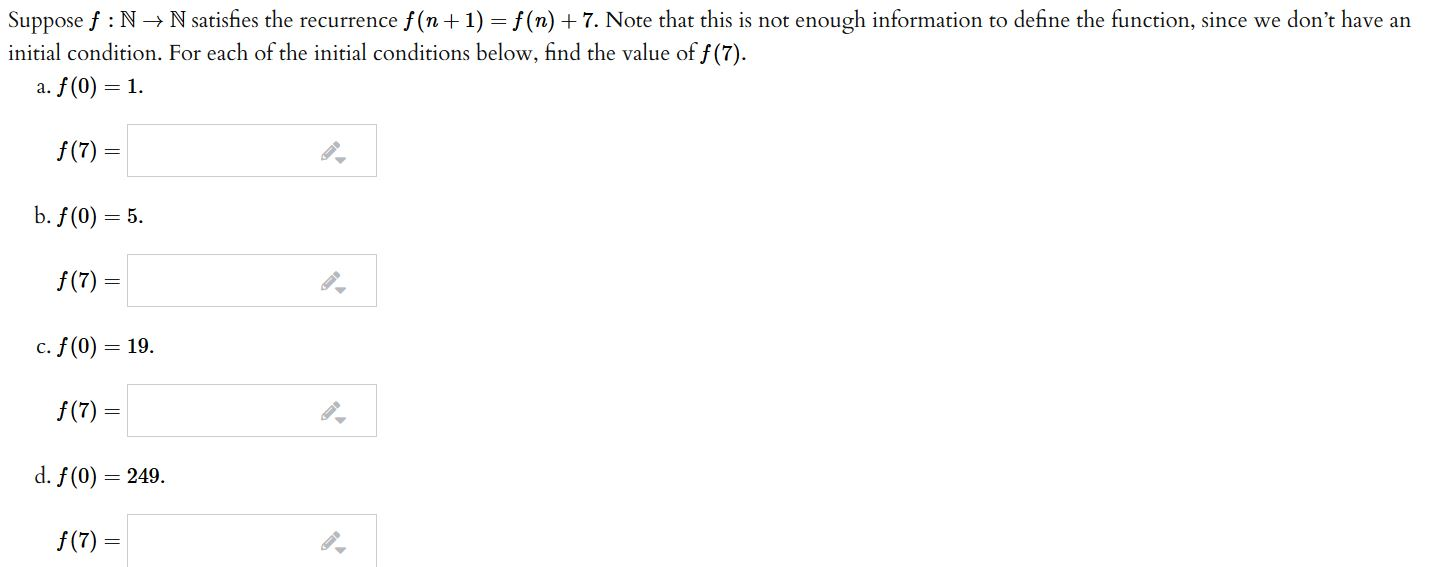Suppose f:N → N satisfies the recurrence f(n+1) = f(n) 7. Note that this is not enough information to define the function, since we don't have an initial condition. For each of the initial conditions below, find the value of f(7). a. f(0) = 1. \$(7) = b. f(0) = 5. f(7) = c. f(0) = 19. f(7) = d. f(0) = 249. f(7) =

• Real analysis 10 11 12 13 please (r 2 4.1 Limit of Function 129 se f: E → R, p is a limit point of E, and li...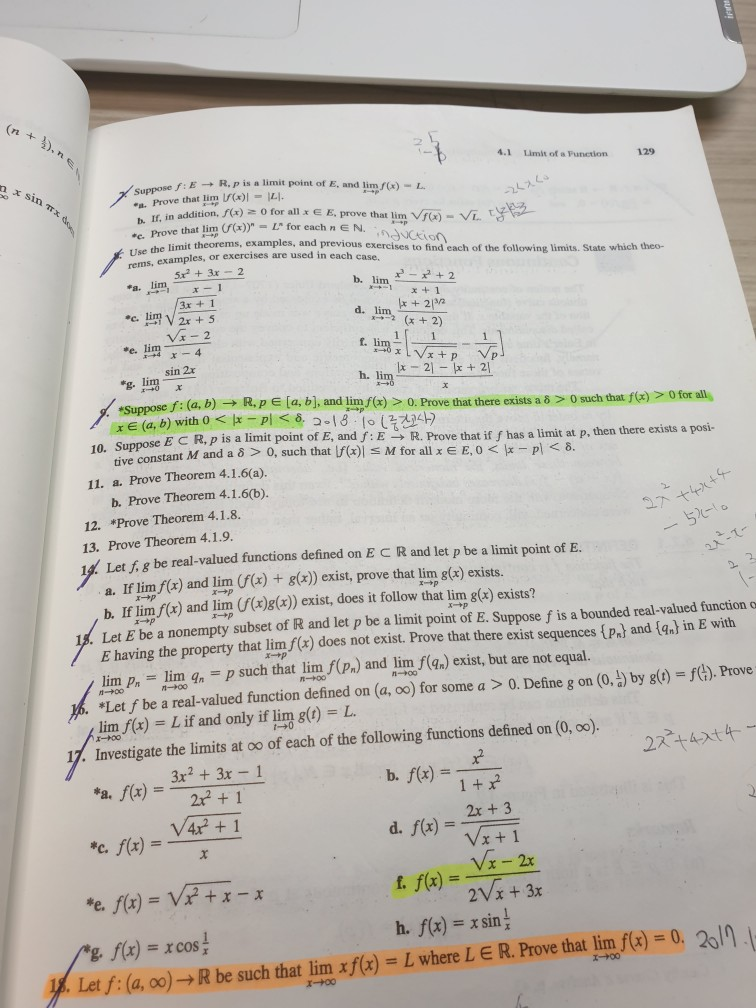Real analysis 10 11 12 13 please (r 2 4.1 Limit of Function 129 se f: E → R, p is a limit point of E, and limf(x)-L. Prove that lim)ILI. h If, in addition, )o for all x E E, prove that lim b. Prove that lim (f(x))"-L" for each n E N. ethe limit theorems, examples, and previous exercises to find each of the following limits. State which theo- rems, examples, or exercises are used in each case....

• Please Answer 135 Below Completely: Definition Let E-R and f : E-+ R be a function....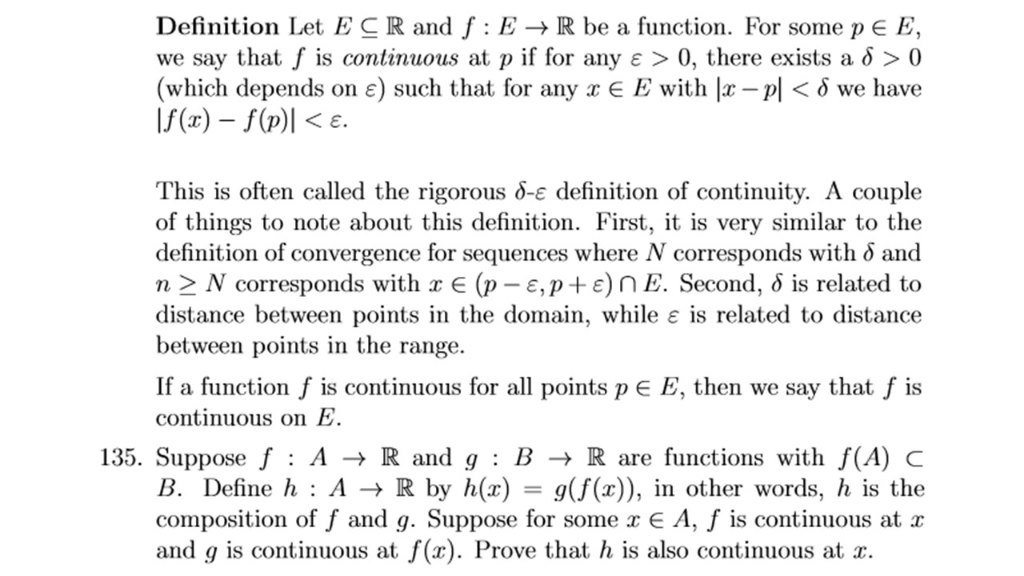Please Answer 135 Below Completely: Definition Let E-R and f : E-+ R be a function. For some p E E' we say that f is continuous at p if for any ε > 0, there exists a δ > 0 (which depends on ε) such that for any x E E with |x-Pl < δ we have If(x) -f(p)le KE. This is often called the rigorous δ-ε definition of continuity. A couple of things to note about this definition....

• Theorem 3.14 Let (neN aand EneN be sequences in R. Let be in R# and suppose that x" → x, y, → oo,...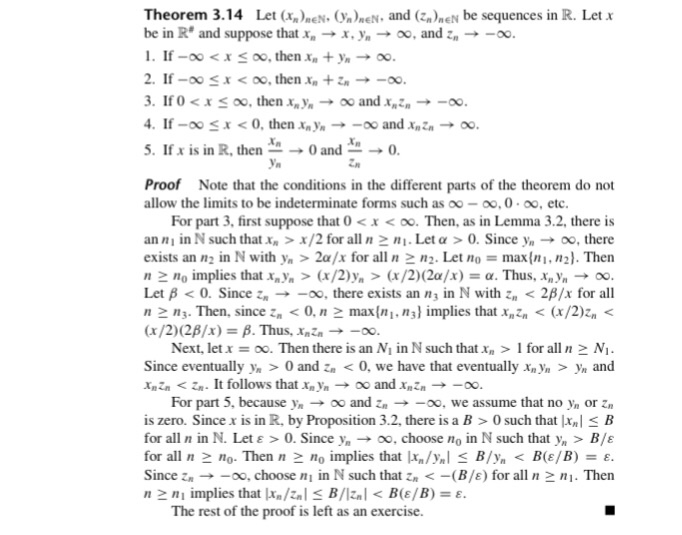Finish the proof of Theorem 3.14. Theorem 3.14 Let (neN aand EneN be sequences in R. Let be in R# and suppose that x" → x, y, → oo, and z" →-oo. . If -oo <x o, then +yn 2. If-oo x < 00, then x" + Zn →-00 4. If-oo x < 0, then xoY" →-00 and xnZn → oo. 5. If x is in R. then-→0and-" →0 Proof Note that the conditions in the different parts of the...

• Implicit Function Theorem in Two Variables: Let g: R2 → R be a smooth function. Set {(z, y) E R2 ...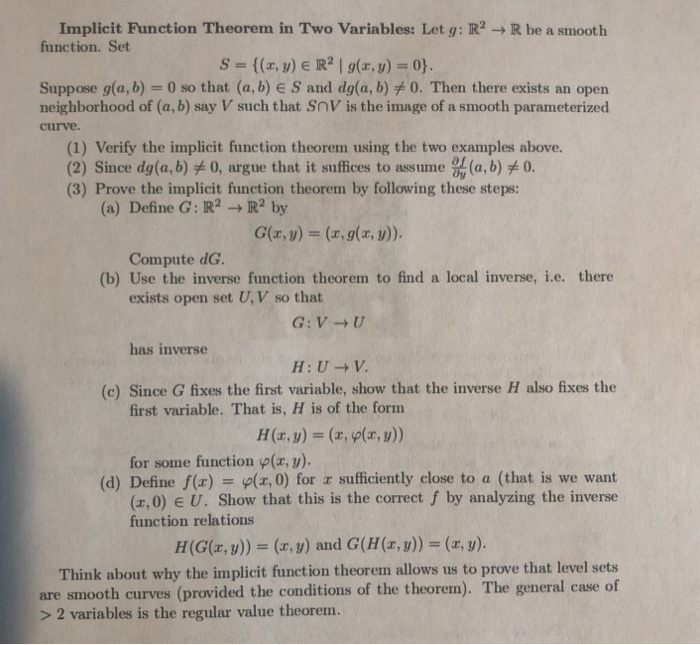Implicit Function Theorem in Two Variables: Let g: R2 → R be a smooth function. Set {(z, y) E R2 | g(z, y) = 0} S Suppose g(a, b)-0 so that (a, b) E S and dg(a, b)メO. Then there exists an open neighborhood of (a, b) say V such that SnV is the image of a smooth parameterized curve. (1) Verify the implicit function theorem using the two examples above. 2) Since dg(a,b) 0, argue that it suffices to...

• 8 marks] Number representations. We define the predicate BT(n,): "r has a balanced ternary repres...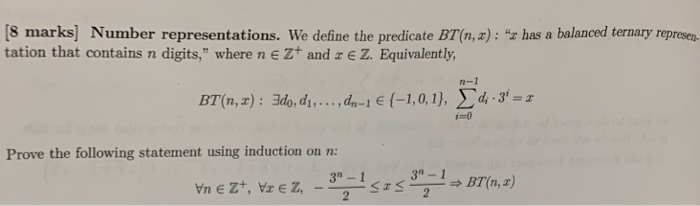8 marks] Number representations. We define the predicate BT(n,): "r has a balanced ternary represen tation that contains n digits," where n eZ+ and z e Z. Equivalently BT(n,z) : 34, di, ,dn-le(-1,0,1), Σdi.gi=z Prove the following statement using induction on n 3a-1 8 marks] Number representations. We define the predicate BT(n,): "r has a balanced ternary represen tation that contains n digits," where n eZ+ and z e Z. Equivalently BT(n,z) : 34, di, ,dn-le(-1,0,1), Σdi.gi=z Prove the following...

• Define a relation < on Z by m <n iff |m| < |n| or (\m| =...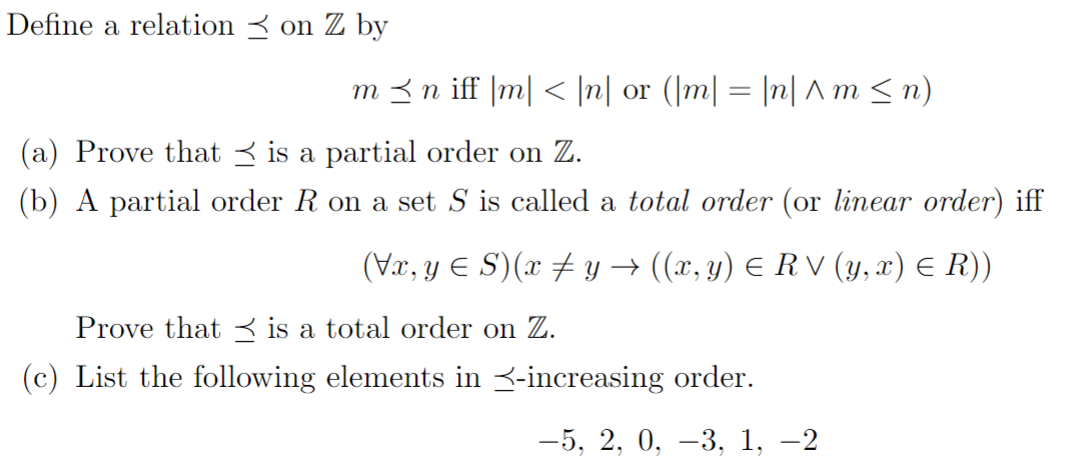Define a relation < on Z by m <n iff |m| < |n| or (\m| = |n| 1 m <n) (a) Prove that < is a partial order on Z. (b) A partial order R on a set S is called a total order (or linear order) iff (Vx, Y ES)(x + y + ((x, y) E R V (y,x) E R)) Prove that is a total order on Z. (c) List the following elements in <-increasing order. –5, 2,...

• Number Theory 13 and 14 please! 13)) Let n E N, and let ā, x, y E Zn. Prove that if ā + x = ā + y, then x-y. 14. In this exercise, you will prove that the additive inverse of any element of Z, is uni...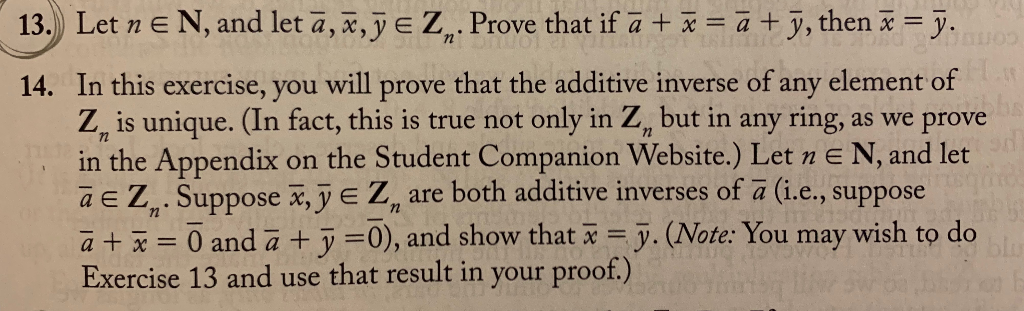Number Theory 13 and 14 please! 13)) Let n E N, and let ā, x, y E Zn. Prove that if ā + x = ā + y, then x-y. 14. In this exercise, you will prove that the additive inverse of any element of Z, is unique. (In fact, this is true not only in Z, but in any ring, as we prove in the Appendix on the Student Companion Website.) Let n E N, and let aE Z...